Request your copy of SimphoSOFT® now

Webinars
Product brochureApplication notesIn many important practical situations, beam propagation through a nonlinear media can be accurately modeled by the following nonlinear Schrödinger equation (NLS)

 i Qη + Δ⊥Q + |Q|2 Q = 0 (NLS)

with a function Q0(ρ) as an incident radially symmetric pulse Q(ρ, η=0)= Q0(ρ) (here Qη = Q / η and the Laplacian Δ is given by  Δ= ∂2/∂ρ2 + (1/ρ)(∂/∂ρ),  η=z / Ldf  is a normalized propagation distance, and ρ= (x2+y2)1/2 / R0 is the normalized distance from the center of the pulse in the transverse two-dimensional plane, Ldf  is the diffraction length and R0 is the beam’s FW1/eM radius).

NLS equation is a paraxial approximation of the following nonlinear Helmholtz (NHL) equation [1,2]

 Ezz(x,y,z,t) + Δ⊥E + (k0)2(1+(2n2/n0)|E|2) E = 0 (NHL)

derived by assuming the following:

• Electric field is written in the following SVEA form, with Q(x,y,z,t) slowly varying along propagation direction z, 0 / 2πR0)2Qηη ≈ 0, which is satisfied when λ0 << R0:  E(x,y,z,t) = R0 k0 (2n2/n0)1/2 Q(x,y,z,t) exp[ -i (ω0t– k0z) ]
• where back-propagation term Q*(x,y,z,t) is excluded

 References  G. Fibich A and S. Tsynkov B, Numerical solution of the nonlinear Helmholtz equation using nonorthogonal expansions, J. Comp. Phys. 210, (2005), 183-224.  G. Baruch, G. Fibich, and Semyon Tsynkov, Simulations of the nonlinear Helmholtz equation: arrest of beam collapse, nonparaxial solitons and counter-propagating beams, Opt. Express 16, 13323-13329 (2008).SimphoSOFT® propagation mathematical model is based on paraxaial approximation.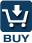SimphoSOFT® can be purchased as a single program and can be also configured with Energy Transfer add-on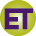, Multi-Beam add-on, Optimization add-on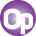, Z-scan add-on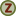, and MPA Info+ add-onfor an additional charge. Please, contact our sales staff for more information e-mail phone: +1 (973) 621-2340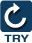You can request a remote evaluation of our software by filling out online form -- no questions asked to get it, no need to install it on your computer.